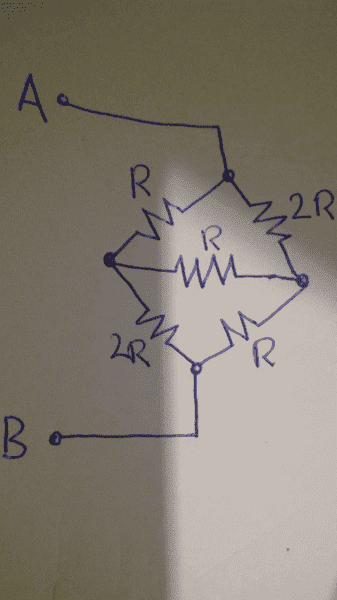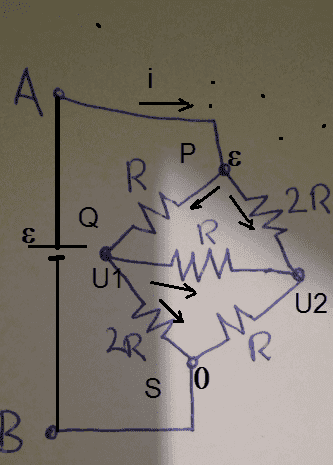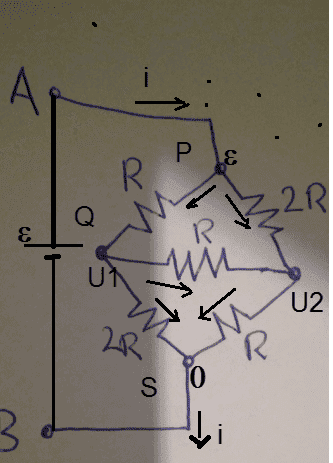# Resistance in circuits

• physicsStudent00

## Homework Statement

1) what is the total resistance of this circuit between points A and B?

2) if the horizontal resistor was replaced with an ammeter what would it read if a 6V battery was placed between points A and B? let R = 20 ohms## The Attempt at a Solution

had multiple attempts, the solution for 1) is 7R/5 but I've never got that, I've been getting 7R/3 and then I'm yet to move to part 2 because I've been getting the resistance for part 1 wrong, which is most likley to be with how I am imagining the current moving through the circuit

Try doing part 2. Working on it may provide insight for part 1.

•physicsStudent00
Try doing part 2. Working on it may provide insight for part 1.
so for part 2 the circuit is split into 2 parallel paths each of equal resistance, therefore the current will be the same in each of the parallel paths, meaning that:
if the total resistance is 30ohms,
I=V/R=6/30=0.2A
so the current in each path is 0.2/2=0.1A

had multiple attempts, the solution for 1) is 7R/5 but I've never got that, I've been getting 7R/3
Have you studied delta- star conversion? That is the key step in solving part 1.

so for part 2 the circuit is split into 2 parallel paths each of equal resistance, therefore the current will be the same in each of the parallel paths,
This is not true. There is an ammeter in place of the horizontal resistance R.

•davenn
Have you studied delta- star conversion? That is the key step in solving part 1.

This is not true. There is an ammeter in place of the horizontal resistance R.
i don't have any idea about a delta-star conversion, so the ammeter would provide a pathway for the current to flow across, also it would have some resistance, so therefore it creates an extra path. the current I am guessing would flow through the ammeter, from the left to the right? as it goes from the path of lowest resistance?

as it goes from the path of lowest resistance?
That is one approach one should give up in circuit analysis. Current flows according to Ohm's law and follows KCL , not caring whether the path is of least resistance or highest resistance.

i don't have any idea about a delta-star conversion
You should look it up. It is a simple and very useful method for simplification of networks.

For part 2, draw the circuit diagram and simplify the network. Find the total current and use current division rule to get the ammeter current.

•davenn
also it would have some resistance, so therefore it creates an extra path
I missed this part in your post. You can assume the ammeter resistance to be zero in case it is not specified. (Even if it is specified, its value is very small).

No need to delta-Y conversion. Imagine you connect a battery of known emf to points A, B, and write the current law for the nodes P, Q, S in terms the potentials U1, U2, and ε assuming zero potential at the negative terminal of the battery.In case b) the ammeter can be considered ideal, that is, of zero internal resistance. That would mean U1=U2, the upper pair of resistors are connected in parallel, and so are the lower pair of resistors. What is the resultant then? What is the input current?

•physicsStudent00
No need to delta-Y conversion. Imagine you connect a battery of known emf to points A, B, and write the current law for the nodes P, Q, S in terms the potentials U1, U2, and ε assuming zero potential at the negative terminal of the battery.

View attachment 212349
In case b) the ammeter can be considered ideal, that is, of zero internal resistance. That would mean U1=U2, the upper pair of resistors are connected in parallel, and so are the lower pair of resistors. What is the resultant then? What is the input current?
with the delta Y conversion it makes a lot more sense now, thank you very much for the help.

with the delta Y conversion it makes a lot more sense now, thank you very much for the help.
Have you solved the problem with the delta-Y conversion? Here is an other approach which uses KCL at the nodes P, Q, S

Node P: I - (ε-U1)/R - (ε-U2)/(2R) = 0
Node Q: (ε-U1)/R - (U1-U2)/R - U1/(2R) = 0
Node S: U1/(2R) + U2/R - I = 0
Solve for I. The total resistance is RT = ε/I.